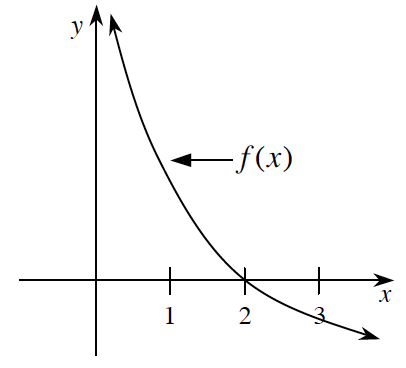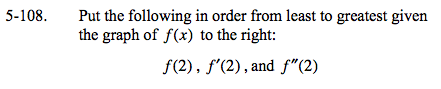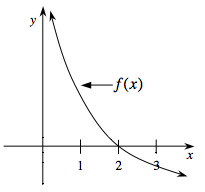### Home > CALC > Chapter 5 > Lesson 5.2.5 > Problem5-108

5-108.
1. Put the following in order from least to greatest given the graph of f(x) below: Homework Help ✎

f(2), f ′(2), and f ″(2)Is f(2) positive, negative or 0?

Is f '(2) positive, negative or 0? Recall that f '(x) represents the slope of f(x).

Is f ''(2) positive, negative or 0? Recall that f ''(x) represents the concavity of f(x).